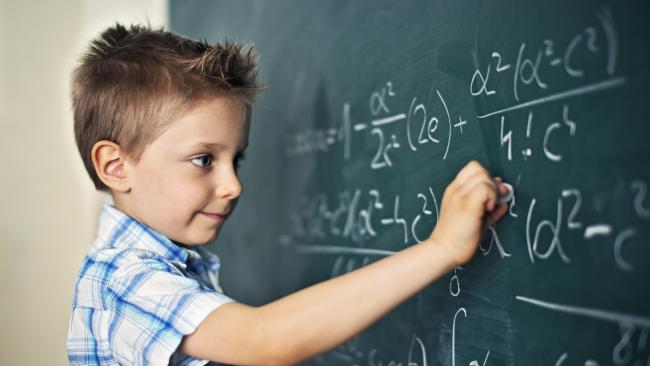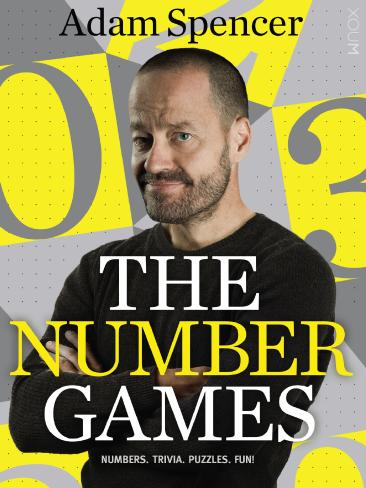Have you tried our 12 Puzzles of Christmas to test your mathematical know-how?

# Can you solve this maths riddle? Year 4 students can

FOR the latest instalment of our 12 Puzzles of Christmas, I'd like to introduce you to a fun type of puzzle invented by fellow numbers nerd Sean Gardiner.

When I say "fun" that doesn't mean it can't be "difficult" … or is that just me?

So far the first four of our 12 Puzzles haven't required much mathematics. Well that changes today! (Test out your brain power with puzzles for day one, day two, day three and day four.)

The maths itself isn't hard - a 4th grader could work out the sums. But discovering the sums we are looking for is considerably harder.

The five numbers have to be placed in the white circles and the four grey circles contain the operators + - x and ÷ in a way that gives a sum with answer 17. Further, we are using the old "order of operations" you might remember from school wherein you do x and ÷ before you do + and -. So 7 + 4 x 5 = 7 + 20 = 27 and 8 - 4 ÷ 2 = 8 - 2 = 6. I've given you some of the operators early on while you warm up, but the last question is a hint-free zone!

So limber up and see if you can figure out where the numbers fit, and which operators they require, to complete the equations above. Too easy? Then see if you can find the unique arrangement that gives the highest "score" in each line, where your score is the 5-digit number you get from reading off the yellow numbers in order from left to right!

SOLUTION BELOWAdam Spencer's new book The Number Games is out now.

7 − 9 ÷ 9 + 6 + 5 = 17

5 − 9 + 5 × 4 + 1 = 17

9 × 8 ÷ 4 − 7 ÷ 7 = 17

9 × 6 − 9 × 5 + 8 = 17

7 + 6 ÷ 9 × 5 × 3 = 17

You might have gotten some slightly different answers, but these give the largest 5 digit numbers, for example 79965 from the first question when 79956 would also work.

Adam Spencer's book The Number Games is available now from all good bookstores or visit